# 3rd Grade Math Lesson on Finding the Area of Figures using Unit Squares

Without overlapping or leaving gaps, cover the geometric figures (squares, rectangles, and irregular figures) with “unit squares” (square cm, square m, square in, square ft). To determine the area of geometric figures, count the number of “unit squares” used to cover the figures.

Lesson Objective: The lesson is aligned to the Common Core State Standards for Mathematics – 3.MD.6 Geometric Measurement – Measure areas by counting unit squares (square cm, square m, square in, square ft).

Materials Required: unit square, graph paper

## Lesson Procedure: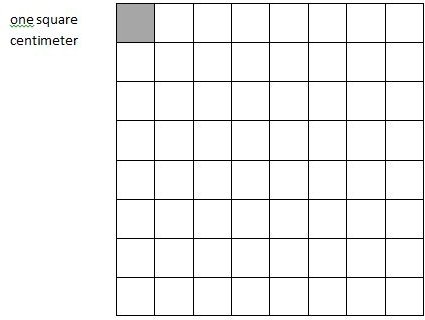Identifying Area of a “Unit Square” as One Square Centimeter

1. Identify the side length of the “unit square” on the graph paper as one centimeter, cm.

2. Identify the area of the “unit square” as one square centimeter, sq cm.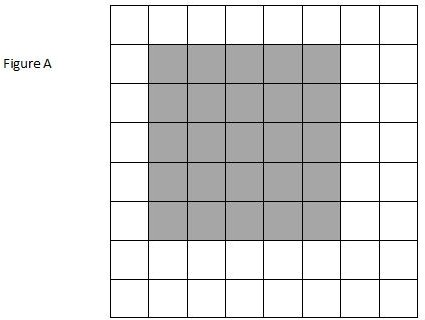3. Count the number of “unit squares” shaded in Figure A.

4. How many “unit squares” are shaded in Figure A?

5. What is the area, in square centimeters, of Figure A?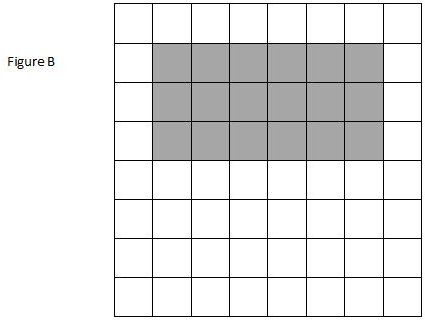6. Count the number of “unit squares” shaded in Figure B.

7. How many “unit squares” are shaded in Figure B?

8. What is the area, in square centimeters, of Figure B?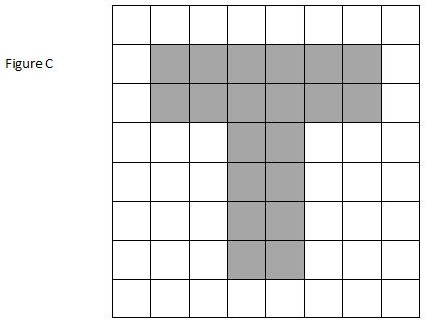9. Count the number of “unit squares” shaded in Figure C.

10. How many “unit squares” are shaded in Figure C?

11. What is the area, in square centimeters, of Figure C?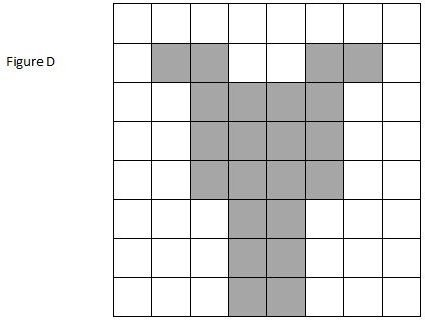12. Count the number of “unit squares” shaded in Figure D.

13. How many “unit squares” are shaded in Figure D?

14. What is the area, in square centimeters, of Figure D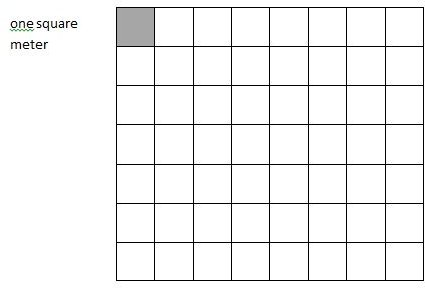Identifying Area of a “Unit Square” as One Square Meter

15. Identify the side length of the “unit square” on the graph paper as one meter, m.

16. Identify the area of the “unit square” as one square meter.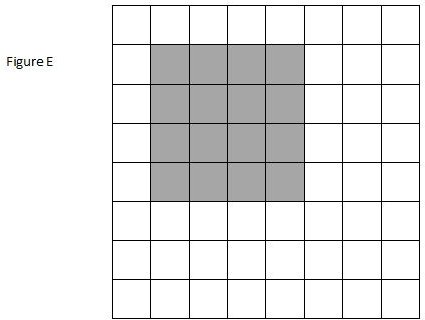17. Count the number of “unit squares” shaded in Figure E.

18. How many “unit squares” are shaded in Figure E?

19. What is the area, in square meters, of Figure E?

4. 25
5. 25 square centimeters
7. 18
8. 18 square centimeters
10. 20
11. 20 square centimeters
13. 22
14. 22 square centimeters
18. 16
19. 16 square centimeters

## Individual or Group Work: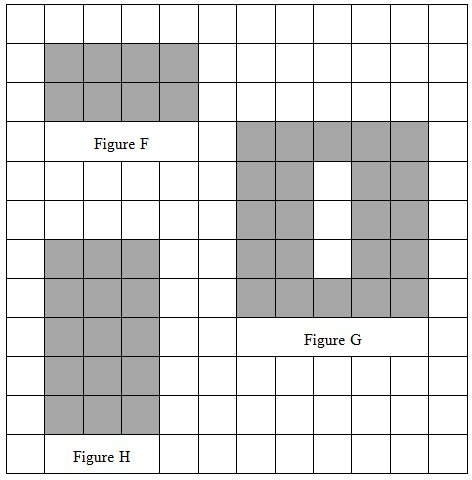1. The area of each “unit square” measures one square meter, sq m.

2. Count the number of “unit squares” shaded in Figures F, G, and H.

3. How many “unit squares” are shaded in Figures F, G, and H?

4. What is the area, in square meters, of Figures F, G, and H?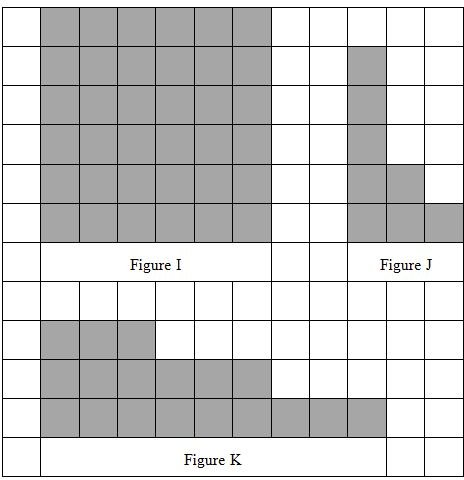5. The area of each “unit square” measures one square inch, sq in.

6. Count the number of “unit squares” shaded in Figures I, J, and K.

7. How many “unit squares” are shaded in Figures I, J, and K?

8. What is the area, in square inches, of Figures I, J, and K?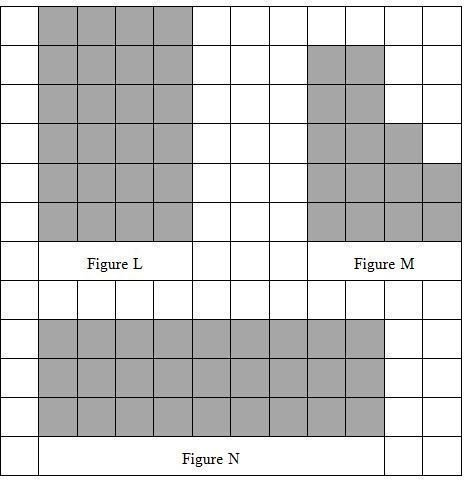9. The area of each “unit square” measures one square foot, sq ft.

10. Count the number of “unit squares” shaded in Figures L, M, and N.

11. How many “unit squares” are shaded in Figures L, M, and N?

12. What is the area, in square feet, of Figures L, M, and N?

3. 8, 22, 15
4. 8 square meters, 22 square meters, 15 square meters
7. 36, 8, 18
8. 36 square inches, 8 square inches, 18 square inches
11. 24, 15, 27
12. 24 square feet, 15 square feet, 27 square feet